# Oneway Anova

The Oneway ANOVA Form does calculations for Fixed and Random Effects Oneway ANOVAs, and the Kruskal-Wallis Nonparametric Oneway ANOVA for ordinal data. With the Fixed Effects model, omega-squared is calculated. With the Random Effects model, the between-component variance, total variance, and Intraclass Correlation coefficient are calculated.

When selected, three dispersion tests will be output: the Bartlett-Box-F, the Levene Test, and the ADM(n-1) procedure.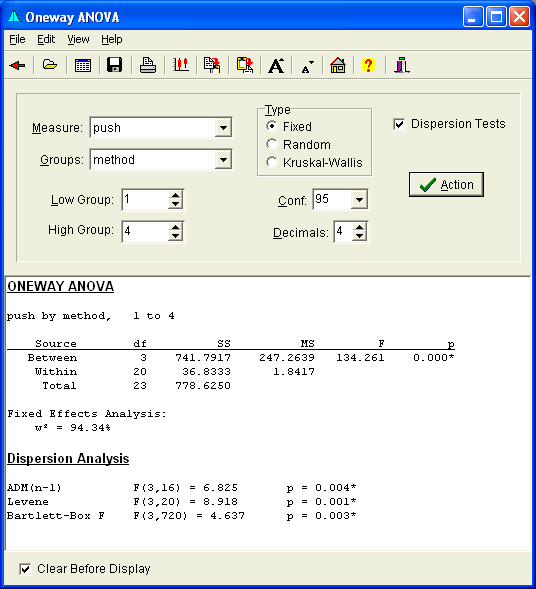## ANOVA Calculations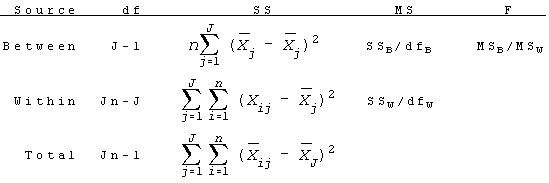Where:

• J is the number of Groups
• n is the sample size per group

### Fixed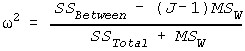Whereis the Omega-Squared value.

### RandomWhere:

•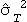is the estimated Treatment Variance, and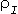is the Intraclass Correlation Coefficient.

### Kruskal-Wallis

The data are ranked, with corrections made for ties. The H statistic is calculated as follows. H' is the correction for ties. H is tested using a Chi-square distribution, with k-1 degrees of freedom.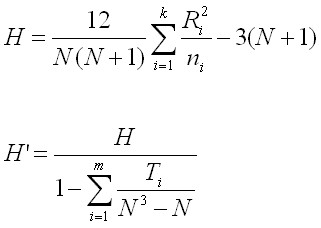## Dispersion Testing

Three dispersion tests are included in MVPstats.

• The ADM(n-1) test is a Oneway on the Absolute Deviations from the Sample Medians, with the center point omitted.
• The Levene test is a Oneway on the Absolute Deviations from the Means.
• The Bartlett-Box-F, which requires Normality, uses the following calculations.Where F has (k-1, f2) degrees of freedom.